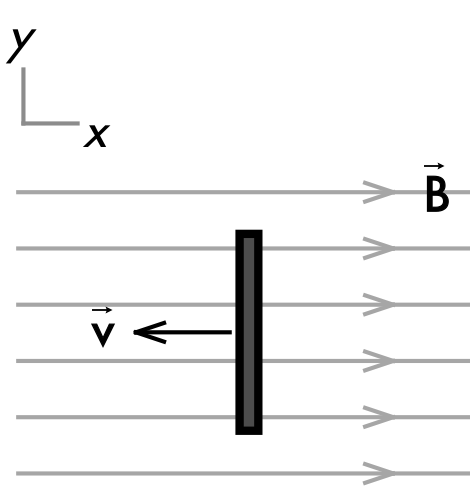## 20180415

### Physics quiz question: higher potential end of moving rod

Physics 205B Quiz 6, spring semester 2013
Cuesta College, San Luis Obispo, CAA conducting rod moves at a constant speed through a uniform magnetic field. The __________ of this rod is at a higher potential.
(A) top.
(B) bottom.
(C) middle.
(D) (None of the above choices, as the entire rod has the same potential.)

Correct answer (highlight to unhide): (D)

Since the angle between the magnetic field and direction of the velocity of the (fictitious) positive (and negative) charges in the rod is 180°, then there is no force exerted on the charges in the rod (as the magnitude of the force depends on the sine of this angle), so no charges are displaced to either end of the rod, and thus there is no potential difference between either end.

Section 30882
Exam code: quiz06h4cK
(A) : 11 students
(B) : 5 students
(C) : 2 students
(D) : 14 students

Success level: 44%
Discrimination index (Aubrecht & Aubrecht, 1983): 0.75